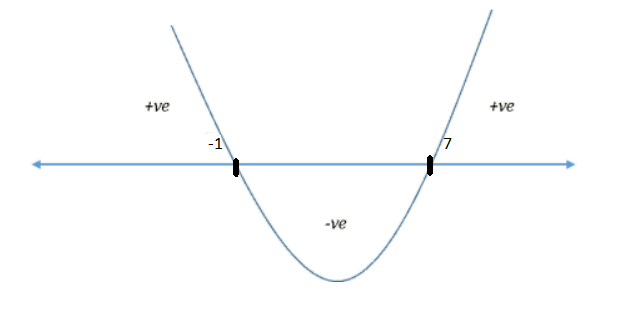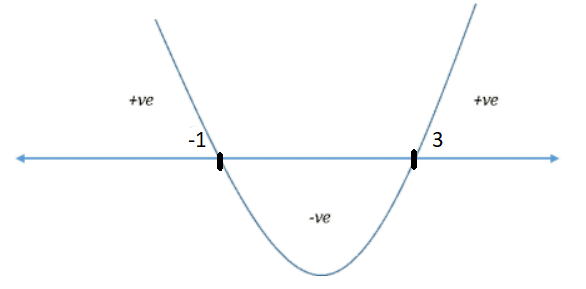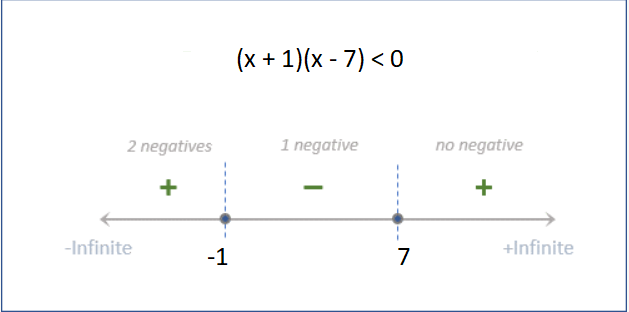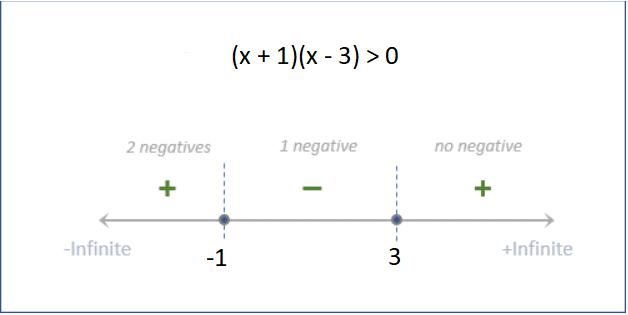GMAT Question of the Day - Daily to your Mailbox; hard ones only

 It is currently 15 Jun 2019, 21:53GMAT Club Daily Prep

Thank you for using the timer - this advanced tool can estimate your performance and suggest more practice questions. We have subscribed you to Daily Prep Questions via email.

Customized
for You

we will pick new questions that match your level based on your Timer History

Track

every week, we’ll send you an estimated GMAT score based on your performance

Practice
Pays

we will pick new questions that match your level based on your Timer History

Not interested in getting valuable practice questions and articles delivered to your email? No problem, unsubscribe here.If x is an integer and it satisfies the inequalities, x^2 – 6x - 7...

Author Message
TAGS:

Hide Tags

e-GMAT RepresentativeD
Joined: 04 Jan 2015
Posts: 2888
If x is an integer and it satisfies the inequalities, x^2 – 6x - 7...  [#permalink]

Show Tags00:00

Difficulty:35% (medium)

Question Stats:76% (01:57) correct24% (01:57) wrongbased on 147 sessions

HideShow timer Statistics

If x is an integer and it satisfies the inequalities, $$x^2 – 6x - 7 < 0$$ and $$2x – x^2 + 3 < 0$$, then which of the following can be the value of x?

A. -1
B. 0
C. 1
D. 2
E. 5

_________________

Originally posted by EgmatQuantExpert on 31 Oct 2018, 04:34.
Last edited by EgmatQuantExpert on 31 Oct 2018, 23:40, edited 1 time in total.
CEOD
Status: GMATINSIGHT Tutor
Joined: 08 Jul 2010
Posts: 2940
Location: India
GMAT: INSIGHT
Schools: Darden '21
WE: Education (Education)
Re: If x is an integer and it satisfies the inequalities, x^2 – 6x - 7...  [#permalink]

Show Tags

EgmatQuantExpert wrote:
If x is an integer and it satisfies the inequalities, $$x^2 – 6x - 7 < 0$$ and $$2x – x^2 + 3 < 0$$, then which of the following can be the value of x?

A. -1
B. 0
C. 1
D. 2
E. 5

$$x^2 – 6x - 7 < 0$$
i.e. $$x^2 – 7x + x - 7 < 0$$

i.e.$$(x-7)*(x+1) < 0$$

Product of two things is less than zero i.e. one of them must be positive and other must be Negative. Also since (X-7) is smaller than (x+1) therefore (X-7) must be negative

i.e. $$(x-7) < 0$$ and $$(x+1) > 0$$

i.e. $$-1 < x < 7$$

Now, $$2x – x^2 + 3 < 0$$

i.e. $$x^2 - 2x - 3 > 0$$

i.e. $$x^2 - 3x +x - 3 > 0$$

i.e. $$(x-3)*(x+1) > 0$$

i.e. $$x > 3 or x < -1$$

Looking at both blue results we can infer that

$$3 < x < 7$$

i.e. x can only be 5 out of given option choices

_________________
Prosper!!!
GMATinsight
Bhoopendra Singh and Dr.Sushma Jha
e-mail: info@GMATinsight.com I Call us : +91-9999687183 / 9891333772
Online One-on-One Skype based classes and Classroom Coaching in South and West Delhi
http://www.GMATinsight.com/testimonials.html

ACCESS FREE GMAT TESTS HERE:22 ONLINE FREE (FULL LENGTH) GMAT CAT (PRACTICE TESTS) LINK COLLECTION
ManagerS
Joined: 04 Jun 2018
Posts: 157
GMAT 1: 610 Q48 V25GMAT 2: 690 Q50 V32GMAT 3: 710 Q50 V36Re: If x is an integer and it satisfies the inequalities, x^2 – 6x - 7...  [#permalink]

Show Tags

While I could solve the question by finding out the range of individual equations and then finding out the common value, I have a doubt.

Why can't I add the equations and still get the same result?

If we were to try add these equations ,we would get:

-4x-4<0
i.e x<-1

Why would this method not work?
I know there is a conceptual gap here because x<-1 is not a valid range.

Can some expert help out?
gmatbusters
EgmatQuantExpert
CEOD
Status: GMATINSIGHT Tutor
Joined: 08 Jul 2010
Posts: 2940
Location: India
GMAT: INSIGHT
Schools: Darden '21
WE: Education (Education)
Re: If x is an integer and it satisfies the inequalities, x^2 – 6x - 7...  [#permalink]

Show Tags

1
nitesh50 wrote:
While I could solve the question by finding out the range of individual equations and then finding out the common value, I have a doubt.

Why can't I add the equations and still get the same result?

If we were to try add these equations ,we would get:

-4x-4<0
i.e x<-1

Why would this method not work?
I know there is a conceptual gap here because x<-1 is not a valid range.

Can some expert help out?
gmatbusters
EgmatQuantExpert

nitesh50

Addition of inequalities is valid for Linear inequalities with same inequation signs.

Here we are dealing with quadratic inequations hence we can not simply add to get the solution

I hope this helps!!!
_________________
Prosper!!!
GMATinsight
Bhoopendra Singh and Dr.Sushma Jha
e-mail: info@GMATinsight.com I Call us : +91-9999687183 / 9891333772
Online One-on-One Skype based classes and Classroom Coaching in South and West Delhi
http://www.GMATinsight.com/testimonials.html

ACCESS FREE GMAT TESTS HERE:22 ONLINE FREE (FULL LENGTH) GMAT CAT (PRACTICE TESTS) LINK COLLECTION
Retired ModeratorV
Joined: 27 Oct 2017
Posts: 1225
Location: India
GPA: 3.64
WE: Business Development (Energy and Utilities)
Re: If x is an integer and it satisfies the inequalities, x^2 – 6x - 7...  [#permalink]

Show Tags

Dear nitesh50

Adding the quadratic inequalities/equations might lead to loss of some roots, and you can get a larger Set as other conditions get lost with the loss of quadratic term.

Please note that there is a mistake in your solution which seems to show invalid range

-4x-4<0
or, -4<4x
or x>-1

note that the actual answer x = 5 also satisfy this condition.

nitesh50 wrote:
While I could solve the question by finding out the range of individual equations and then finding out the common value, I have a doubt.

Why can't I add the equations and still get the same result?

If we were to try add these equations ,we would get:

-4x-4<0
i.e x<-1

Why would this method not work?
I know there is a conceptual gap here because x<-1 is not a valid range.

Can some expert help out?
gmatbusters
EgmatQuantExpert

_________________
ManagerS
Joined: 04 Jun 2018
Posts: 157
GMAT 1: 610 Q48 V25GMAT 2: 690 Q50 V32GMAT 3: 710 Q50 V36Re: If x is an integer and it satisfies the inequalities, x^2 – 6x - 7...  [#permalink]

Show Tags

gmatbusters wrote:
Dear nitesh50

Adding the quadratic inequalities/equations might lead to loss of some roots, and you can get a larger Set as other conditions get lost with the loss of quadratic term.

Please note that there is a mistake in your solution which seems to show invalid range

-4x-4<0
or, -4<4x
or x>-1

note that the actual answer x = 5 also satisfy this condition.

nitesh50 wrote:
While I could solve the question by finding out the range of individual equations and then finding out the common value, I have a doubt.

Why can't I add the equations and still get the same result?

If we were to try add these equations ,we would get:

-4x-4<0
i.e x<-1

Why would this method not work?
I know there is a conceptual gap here because x<-1 is not a valid range.

Can some expert help out?
gmatbusters
EgmatQuantExpert

Hi gmatbusters
Thank you for correcting my mistake.
this might be a stupid question, but
Why is that when we are adding 2 inequalities, it leads to loss of a certain range.
I guess What I am still looking for is a bit of a conceptual understanding to it.

Regards
Nitesh
Retired ModeratorV
Joined: 27 Oct 2017
Posts: 1225
Location: India
GPA: 3.64
WE: Business Development (Energy and Utilities)
Re: If x is an integer and it satisfies the inequalities, x^2 – 6x - 7...  [#permalink]

Show Tags

1
Hi

See this example

if x^2 = 2x
and we cancel out x from both sides ( it leads to loss of root, x = 0)
We get x = 2.

Whereas,
x^2 = 2x
or, x(x-2) = 0
or x = 0 or 2

By cancelling some terms you are loosing roots.

nitesh50 wrote:
gmatbusters wrote:
Dear nitesh50

Adding the quadratic inequalities/equations might lead to loss of some roots, and you can get a larger Set as other conditions get lost with the loss of quadratic term.

Please note that there is a mistake in your solution which seems to show invalid range

-4x-4<0
or, -4<4x
or x>-1

note that the actual answer x = 5 also satisfy this condition.

nitesh50 wrote:
While I could solve the question by finding out the range of individual equations and then finding out the common value, I have a doubt.

Why can't I add the equations and still get the same result?

If we were to try add these equations ,we would get:

-4x-4<0
i.e x<-1

Why would this method not work?
I know there is a conceptual gap here because x<-1 is not a valid range.

Can some expert help out?
gmatbusters
EgmatQuantExpert

Hi gmatbusters
Thank you for correcting my mistake.
this might be a stupid question, but
Why is that when we are adding 2 inequalities, it leads to loss of a certain range.
I guess What I am still looking for is a bit of a conceptual understanding to it.

Regards
Nitesh

_________________
e-GMAT RepresentativeD
Joined: 04 Jan 2015
Posts: 2888
If x is an integer and it satisfies the inequalities, x^2 – 6x - 7...  [#permalink]

Show Tags

Solution

Given:
• We are given that x is an integer, and
• We are also given two inequalities,
o $$x^2 – 6x - 7 < 0$$
o $$2x – x^2 + 3 < 0$$

To find:
• We need to find out which among the given answer choices can be a possible value of x that satisfies the given information

Approach and Working:
• We need to solve both the quadratic inequalities to arrive at the answer.
• Let’s solve the first inequality, $$x^2 - 6x – 7 < 0$$
o Implies, (x - 7)(x + 1) < 0

Approach 1: Wavy-line method• Thus, the expression is negative for -1 < x < 7
• The values of x that satisfy this inequality are, x = {0, 1, 2, 3, 4, 5, 6}

Now, let’s solve the second inequality, $$2x - x^2 - 3 < 0$$
o Multiplying by -1, we get, $$x^2 – 2x - 3 > 0$$
o Implies, (x - 3)(x + 1) > 0• Thus, the expression is positive for x < -1 and x > 3

Combining both the results, -1 < x < 7, and (x < -1 or x > 3), we can say that the values of x that satisfy this inequality are, 3 < x < 7
• Since, x is an integer, x = {4 , 5 , 6}

Approach 2: Number-line method

Number- line for the expression (x + 1)(x - 7) < 0 is,• Thus, the expression is negative for -1 < x < 7

Now, let’s draw the number-line for the expression, (x + 1)(x - 3) > 0• Thus, the expression is positive for x < -1 or x > 3

Combining both these results, we get, 3 < x < 7
• Since, x is an integer, the values of x that satisfy the given expression are {4, 5, 6}

Hence, the correct answer is option E.

_________________
Senior ManagerS
Joined: 12 Sep 2017
Posts: 262
If x is an integer and it satisfies the inequalities, x^2 – 6x - 7...  [#permalink]

Show Tags

GMATinsight wrote:
EgmatQuantExpert wrote:
If x is an integer and it satisfies the inequalities, $$x^2 – 6x - 7 < 0$$ and $$2x – x^2 + 3 < 0$$, then which of the following can be the value of x?

A. -1
B. 0
C. 1
D. 2
E. 5

$$x^2 – 6x - 7 < 0$$
i.e. $$x^2 – 7x + x - 7 < 0$$

i.e.$$(x-7)*(x+1) < 0$$

Product of two things is less than zero i.e. one of them must be positive and other must be Negative. Also since (X-7) is smaller than (x+1) therefore (X-7) must be negative

i.e. $$(x-7) < 0$$ and $$(x+1) > 0$$

i.e. $$-1 < x < 7$$

Now, $$2x – x^2 + 3 < 0$$

i.e. $$x^2 - 2x - 3 > 0$$

i.e. $$x^2 - 3x +x - 3 > 0$$

i.e. $$(x-3)*(x+1) > 0$$

i.e. $$x > 3 or x < -1$$

Looking at both blue results we can infer that

$$3 < x < 7$$

i.e. x can only be 5 out of given option choices

Good night GMATinsight

I understood the whole method but I am struggling with on doubt:

i.e.$$(x-7)*(x+1) < 0$$

If I want to solve it algebraically, isn't the following correct?

x < 7
x < 1

What am I doing wrong?, I mean I understand the concept but why does the < sign change here if we are just adding/subtracting?

Kind regards!If x is an integer and it satisfies the inequalities, x^2 – 6x - 7...   [#permalink] 15 Jan 2019, 21:03
Display posts from previous: Sort by

If x is an integer and it satisfies the inequalities, x^2 – 6x - 7...